### John L. Sabo and David M. Post. 2008. Quantifying periodic, stochastic, and catastrophic environmental variation. Ecological Monographs 78:19–40.

Appendix A. Extreme event tutorial.

Estimation of extreme event statistics via Pearson Type III function

Extreme statistics are estimated from a series of annual extreme observations as :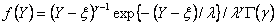(A.1)

where, Y is an annual extreme observation (Ha or La) and the Pearson type-III function is described by three parameters, a mean (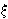), a standard deviation (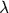) and the coefficient of skewness (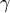).   From this distribution, we estimated the T-year high flow (Y~Ha) or low flow (Y~La)events as: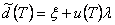(A.2)

whereandare estimated by the mean and standard deviation, respectively of the annual extremes (i.e.,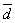and sd) and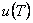is a frequency factor whose estimator is related to the probability of non-exceedance of a given event (1–1/T) and coefficient of skewness via the gamma distribution. We then estimated the coefficient of skewness as: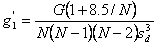(A.3a)

where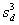is the cube of the standard deviation of annual extremes and,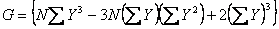(A.3b)

Frequency factors () for 2- and 10-year high and low flows were computed from Ha and La and used with estimates of the mean and variance of high- and low-flow series to estimate 7-day, 2-year, and 10-year high and low flows using tabled values (Haan 1977).

LITERATURE CITED

Haan, C. T. 1977. Statistical Hydrology. Iowa State University Press, Ames, Iowa, USA.

[Back to M078-002]